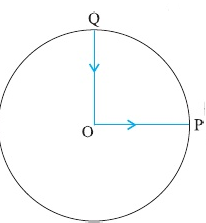Question

Solution

Correct option is

24 ms–1

Let the total journey be 2x metres. A distance x is covered with a speed of 20ms–1 and remaining distance x with a speed of 30ms–1. Therefore, total time taken isSIMILAR QUESTIONS

Q1A cyclist starts from the centre O of a circular track of radius r = 1 km, reaches the edge P of the track and then cycles along circumference and stops at point Q. if the total time take is 10 min, what is the average velocity of the cyclist?

Q2

Give the speed-time graph of the motion of a car. What is the ratio of the distance travelled by the during the last two seconds to the total distance travelled in seven seconds?Q3

A stone falls from rest. The distance covered by the stone in the last second of its motion equals the distance covered by it during the first three seconds of its motion. How long does the stone take to reach the ground?

Q4

Two balls of masses m1 and m2 are thrown vertically upward with the speed u. If air resistance is neglected, they will pass through their point of projection in the downward direction with a speed

Q5

The displacement y (in metres) of a body varies with time t (in seconds) asHow long does the body take to come to rest?

Q6

A body goes from A to B with a velocity of 40 kmh–1 and returns from Bto A with a velocity of 60 kmh–1. What is the average velocity of the body during the whole journey?

Q7

The area under the velocity-time graph between any two instants t = t1and t = t2 gives the distance covered in timeQ8

A particle is given a displacement of 4 m in the x-y plane. If the x-component of the displacement vector is 2 m, the y-component will be

Q9

A car, starting from rest, accelerates at a constant rate of 5 ms–2 for some time. It then retards at a constant rate of 10 ms–2 and finally comes to rest. If the total taken is 6 s, What is the maximum speed attained by the car?

Q10

A body, starting from rest, moves in a straight line with a constant acceleration a for a time interval t during which it travels a distance s1. If continues to move with the same acceleration for the next time interval tduring which it travels a distance s2. The relation between s1 and s2 is